## research papersJOURNAL OFSYNCHROTRONRADIATION
ISSN: 1600-5775
Volume 28| Part 5| September 2021| Pages 1437-1443

## Design of reflective phase retarders in the extreme ultraviolet based on chirped Mo/Si multilayer mirrors

aCollege of Mathematics and Physics, Beijing University of Chemical Technology, Chaoyang District North Third Ring Road 15, Beijing 100029, People's Republic of China, and bSchool of Materials Science and Technology, China University of Geosciences, Haidian District Xueyuan Road 29, Beijing 100083, People's Republic of China
*Correspondence e-mail: cylin@mail.buct.edu.cn

(Received 21 December 2020; accepted 5 July 2021; online 13 August 2021)

The extreme ultraviolet (EUV) phase retarder is an important optical element for polarization analysis and conversion of EUV synchrotron radiation. In this paper, a linearly chirped Mo/Si multilayer mirror is used to design an EUV phase retarder. With increasing thickness variation of the chirped multilayer, the reflective phase retardation between s- and p-polarized light increases at first and then reaches its maximum value. When the bilayer number increases from 2 to 20, the maximum phase retardation for an EUV source with a photon energy of 90 eV increases from 5.97° to 245.10° for a linearly chirped Mo/Si multilayer with 14.7 nm central thickness. In addition, the phase retardations of chirped mirrors at different photon energies (80 eV, 85 eV and 90 eV) are also investigated and compared. Furthermore, the physical mechanism of reflective phase retardation improvement is also studied by investigating the field intensity distributions inside chirped mirrors.

### 1. Introduction

With the rapid development of extreme ultraviolet (EUV) optics in recent years, EUV multilayers have received more and more attention due to their flexible spectrum and phase control capabilities for EUV sources (Belyakov & Dmitrienko, 1989; Wang et al., 2006c; Uschakow et al., 2013). In order to accurately analyze the polarization state of EUV synchrotron radiation (Poldi et al., 2020), an EUV phase retarder that can introduce a retardation between the two orthogonal components (s- and p-polarization) of the electric field is necessary (Fonzo et al., 1994; Schäfers et al., 1999). In particular, an EUV phase retarder achieving 90° phase retardation (i.e. quarter-wave plate) can realize the polarization state conversion between linear polarization and the circular polarization of an EUV light source, and achieve nearly 100% degree of circular polarization by choosing a suitable azimuthal angle (Tang et al., 2017), which is widely applied in EUV magnetic circular dichroic imaging (MCD) (Chen et al., 1990) and resonant magnetic scattering (Kao et al., 1994).

In 1990, the reflective and transmissive phase retardation between s- and p-polarized electric field components induced by a periodic Mo/Si multilayer structure was calculated (Kortright & Underwood, 1990). Researchers indicated that a large phase retardation can be formed by a transmissive periodic multilayer near its Bragg peak in the EUV range (MacDonald et al., 2008, 2009; Apfel, 1982), which demonstrated the potential EUV multilayer application as a transmissive phase retarder. Afterwards, the transmissive multilayers were successfully prepared (Kortright et al., 1992; Nomura et al., 1992; Yamamoto et al., 1992) and used as polarization analyzers to measure the phase retardation of soft X-rays. Then, a transmissive multilayer based soft X-ray polarizer was used (Kimura et al., 1992, 1995; Schäfers et al., 1999) to perform a complete polarization measurement of EUV synchrotron radiation. Recently, researchers (Wang et al., 2007) used a numerical method to design a broadband EUV phase retarder and fabricated it on a silicon nitride film by DC magnetron sputtering.

When using an EUV multilayer as a transmissive phase retarder, the EUV light needs to pass through the multilayer. Considering the larger absorption of materials in the EUV region, the substrate of the multilayer needs to be corroded (Lin et al., 2015a) and a thin supporting film is sometimes required to obtain the highest possible transmission output, which make the preparation of a transmissive phase retarder difficult in the EUV region (Xie et al., 2013). A reflective-type phase retarder can avoid substrate corrosion and supporting film preparation, and facilitate the achievement of a high-efficiency EUV phase retarder. However, it was demonstrated that large phase retardation is difficult to achieve by a traditional EUV multilayer mirror with periodic structure (Kortright & Underwood, 1990).

A multilayer with an aperiodic structure possesses more designable parameters than a periodic one, and has been widely used in the design of reflective EUV optical components, such as wide-angle analyzers (Lin et al., 2015a), broadband and narrow-band mirrors (Feigl et al., 2006; Pirozhkov & Ragozin, 2015), and broadband polarizers (Wang et al., 2006b). Recently, it was demonstrated that a reflective EUV phase retarder can be realized by designing structural parameters of an aperiodic multilayer at specific incident angles (Wang et al., 2006a). However, the design of an aperiodic multilayer generally needs a global optimization method and the optimization process is time-consuming, especially for the case of a multilayer with a large number of layers.

The chirped multilayer mirror is a structure whose thickness distribution is regularly varied. It has been widely used for the phase control of ultrashort pulses, such as pulse compression of attosecond pulses (Guggenmos et al., 2018; Morlens et al., 2005; Hofstetter et al., 2011) and dispersion control of femtosecond pulses (Pervak et al., 2007, 2009; Miranda et al., 2011). In this paper, a linearly chirped Mo/Si multilayer is used to design a phase retarder in the EUV region. By optimizing the parameters of a linearly chirped multilayer, the EUV phase retarder achieved various phase retardations with high reflectivity for both polarized lights.

### 2. Theory

#### 2.1. Linearly chirped multilayer

Fig. 1shows the structure of a linearly chirped Mo/Si multilayer, where θ is the angle of incidence, N is the total bilayer number of the chirped Mo/Si multilayer, and D1 and DN represent the thickness of the top and bottom Mo/Si bilayer, respectively. The expression of thickness of the Mo/Si bilayer in the chirped multilayer (DS) is as follows,where Dc represents the central thickness value and ΔD denotes the thickness variation of the Mo/Si bilayer. S represents the order of the bilayer which changes between 1 and N. According to equation (1), the bilayer thickness increases linearly from top bilayer to bottom, and its value increases from D1 = DcΔD to DN = Dc + ΔD.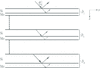Figure 1 Scheme of proposed linearly chirped Mo/Si multilayer.

#### 2.2. Reflectivity and phase

For a multilayer structure, the transmission matrix method is usually used to theoretically analyze its optical performance, such as reflectivity and phase. The characteristic matrix for a layer in the multilayer is written as follows,In equation (2), δi = 2πnidicosθi/λ (i = 1 − N), and ni and di are the complex refractive index and thickness of each layer, respectively. θi is the angle of light in each layer and λ is the wavelength of incident light. ηi = nicosθi for s-polarized incident light, whereas ηi = ni/cosθi for p-polarized. The optical constants of Mo and Si used in the simulation were derived from Henke et al. (1993).

The total characteristic matrix for the whole Mo/Si multilayer isBased on the Fresnel equations, the reflection coefficient r isThe reflectivity R of the multilayer can be calculated usingFor the incident light reflected by the Mo/Si multilayer, the reflective phase φs,p (Kortright & Underwood, 1990) can be written aswhere φs and φp are the phases of reflected s- and p-polarized light, respectively. So, the reflective phase retardation Δφ between the two polarization components of the reflected light can be calculated,In order to quantitatively evaluate the efficiency of a reflective EUV phase retarder designed by the proposed chirped mirror, a parameter called the output efficiency P (Lin et al., 2015b) is employed,Rs and Rp represent the reflectivity of the linearly chirped mirror for s- and p-polarized incident light, respectively.

### 3. Results and discussion

In our simulations, EUV radiation with a photon energy of 90 eV is considered as the incident light. The incident angle is set to be 60° according to our previous research (Lin et al., 2015b), which compared the reflective phase retardation and output efficiency of an aperiodic Mo/Si multilayer under different incident angles, and showed that an incident angle of 60° is beneficial for obtaining a higher output efficiency when realizing a phase retardation of 90°. The central thickness Dc is fixed to 14.7 nm for the linearly chirped Mo/Si multilayer mirror to ensure large reflective phase retardation at 90 eV. The thickness ratio γ is set to 1/3 for each bilayer. The curves of reflective phase retardation Δφ versus thickness variation ΔD for proposed Mo/Si multilayer mirrors with a varying bilayer number N are shown in Fig. 2.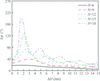Figure 2 Δφ of chirped Mo/Si multilayer mirrors with changing ΔD (θ = 60°, E = 90 eV, N = 6, 9, 12, 15, 18).

As a special case, the results of the periodic Mo/Si multilayer mirrors (ΔD = 0) are also shown in Fig. 2for comparison. With increasing bilayer number N, the reflective phase retardation Δφ0 for a periodic Mo/Si multilayer increases at first, then tends to a constant. The maximum value of Δφ0 is 36.88° for the periodic multilayer with N = 18. The result indicates that it is difficult to realize large reflective phase retardation in the EUV region for a periodic Mo/Si multilayer.

However, for a linearly chirped Mo/Si multilayer mirror with ΔD ≠ 0, the reflective phase retardation Δφ increases from Δφ0 to its maximum value Δφmax, and then decreases in an oscillating way. The results demonstrated that the introduction of chirped thickness variation in an Mo/Si multilayer can significantly improve its reflective phase retardation. In Table 1, Δφmax of optimized chirped mirrors with different N and their corresponding values of ΔD, Rs and Rp are exhibited. When N increases from 6 to 18, Δφmax increases from 21.03° to 203.23°.

 Table 1Δφmax of optimized chirped mirrors with different N and their corresponding values of ΔD, Rs and Rp
 N is the number of cycles, Δφmax is the maximum value of phase retardation, ΔD is the thickness variation value, and Rs and Rp represent the reflectivity for s- and p-polarized light, respectively.
N Δφmax (°) ΔD (nm) Rs Rp
6 21.03 1.71 0.6122 0.3486
9 44.66 3.09 0.4873 0.2156
12 85.21 2.67 0.3553 0.1196
15 161.33 2.11 0.2994 0.0618
18 203.23 1.65 0.3331 0.0458

The reflectivity of chirped Mo/Si multilayer mirrors for s- and p-polarized light with changing ΔD are also investigated, and the results are shown in Fig. 3. For the multilayer with ΔD = 0 (i.e. periodic multilayer), Rs and Rp are both at maximum values. When ΔD increases, Rs and Rp both decrease at first, although phase retardation increases. It seems that there is a trade-off between phase retardation and reflectivity. Fortunately, when ΔD changes in a small range, the chirped mirror maintains high reflection (shown in Table 1) as a periodic multilayer due to its approximate periodic structure which helps reflectance superimpose near its Bragg peak.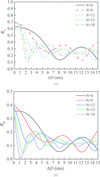Figure 3 Reflectivity of chirped Mo/Si multilayer mirrors for (a) s-polarized and (b) p-polarized light with changing ΔD.

To further investigate the maximum achievable reflective phase retardation and output efficiency of a chirped Mo/Si multilayer mirror (Δφmax and Pcm) with different bilayer number N, we plot the curves of Δφmax and Pcm of the chirped multilayer with changing N in Fig. 4. The maximum reflective phase retardation and output efficiency of the periodic multilayer (Δφ0 and Ppm) under the same conditions is also exhibited for comparison. When N increases from 2 to 20, Δφmax increases from 5.97° to 245.10°, while Δφ0 only increases from 5.97° to 36.88°. In addition, Pcm and Ppm both increase at first and then decrease with increasing N, but Pcm decreases more sharply when N is larger than 6. We found that although the maximum reflective phase retardation of a chirped multilayer increases drastically with increasing N, its output efficiency also decreases sharply, indicating the trade-off between these two parameters.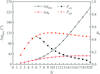Figure 4 Maximum reflective phase retardation and output efficiency of chirped and periodic Mo/Si multilayer mirrors with different N values. Δφmax and Δφ0 represent the maximum values of the phase retardation of the chirped multilayer and periodic multilayer, respectively; Pcm and Ppm represent the output efficiency of the two structures.

To further study the performance of optimized chirped mirrors that can realize maximum reflective phase retardation Δφmax, the corresponding values of ΔD, Rs and Rp with changing N are exhibited in Fig. 5. In Fig. 5(a), with increasing N, Rs and Rp both present an oscillating variation, and both show their maximum at N = 6. Fig. 5(b) shows that ΔD which realizes Δφmax increases at first and then decreases with increasing N. It should be noted that the ΔD for realizing Δφmax varies in thickness only slightly from 0 to 3.5 nm, which is considered to be the reason for high reflectivity as shown in Fig. 5(a).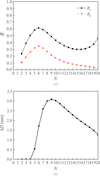Figure 5 Corresponding values of (a) Rs and Rp, and (b) ΔD, of the optimized chirped mirrors with changing N.

Furthermore, the linearly chirped mirrors are optimized for different photon energies (E = 80 eV, 85 eV and 90 eV) of incident light, and the maximum phase retardation Δφmax of the chirped Mo/Si multilayer with different values of N is shown in Fig. 6. The value of Δφmax increases with N of the multilayer for 80, 85 and 90 eV. Phase retardation from 0° to 180° is realized for each photon energy. However, it seems that, at lower photon energies, the linearly chirped mirror can achieve a higher Δφmax.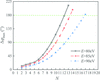Figure 6 Maximum phase retardation Δφmax of chirped Mo/Si multilayer with different values of N under different photon energies.

To further compare the reflective performance of the optimized phase retarder at different photon energies, we list the performance parameters of quarter-wave plates (Δφ = 90°) and half-wave plates (Δφ = 180°) attributed to the linearly chirped Mo/Si multilayer in Table 2. In order to realize quarter-wave or half-wave plates, the linearly chirped multilayer needs a higher N but smaller ΔD at a larger photon energy. In addition, the corresponding values of P all increase with E due to the smaller absorption coefficient of Mo at larger E. In particular, for an incident light with a 90 eV photon energy, an optimized chirped mirror can realize 16.65% or 7.04% output efficiency when designed as a quarter-wave plate or a half-wave plate, respectively. When a chirped multilayer structure based quarter-wave plate is used for the polarization conversion of monochromatic EUV light with linear polarization, nearly 100% degree of circular polarization can be achieved by adjusting the azimuthal angle α between the incident light and the plate to fulfill α = arctan(|rp/rs|) (Tang et al., 2017), such as 17.97° for 80 eV, 20.64° for 85 eV and 24.71° for 90 eV.

 Table 2Performance parameters of optimized phase retarders
 E is the photon energy, Dc is the central thickness value, N is the number of cycles, ΔD is the thickness variation value, Δφ is the achieved phase retardation, Rs and Rp represent the reflectivity for s- and p-polarized light, respectively, and P is the output efficiency.
E (eV) Dc (nm) N ΔD (nm) Δφ (°) Rs Rp P
80 17.6 10 3.53 89.97 0.4137 0.0435 0.0787
13 2.69 182.75 0.4163 0.0011 0.0022
85 15.8 11 2.1 90.03 0.4921 0.0698 0.1223
14 1.67 180.54 0.4581 0.0070 0.0138
90 14.7 13 1.94 89.95 0.4765 0.1009 0.1665
17 1.69 180.19 0.3639 0.0390 0.0704

We studied the physical mechanism of phase retardation improvement induced by a linearly chirped multilayer mirror. The field intensity (|E|2) distribution inside an optimized chirped Mo/Si multilayer mirror with 13 bilayers (ΔD = 1.94 nm, Dc = 14.7 nm) at 90 eV as in Table 2is shown in Fig. 7(a). S represents the order of the bilayer which changes between 1 and N. The field distribution of a periodic multilayer (ΔD = 0 nm, Dc = 14.7 nm) whose total thickness is the same as the chirped mirror is also shown in Fig. 7(b) for comparison. We observed that the maximum field intensity of the s- and p-polarized light inside the chirped mirror appears at a greater distance than those inside the periodic multilayer, indicating that the light travels deeper inside the multilayer. The further the s- and p-polarized light travel, the more interfaces they encounter and the larger phase retardation they obtain, which is considered to be the origin of reflective phase retardation improvement induced by a chirped multilayer mirror. However, when the EUV light goes further inside the multilayer, the material absorption becomes larger, leading to a low reflectivity for s- or p-polarized light and finally causes low output efficiency. Nevertheless, the reflectivity and output efficiency can be still further improved by optimizing the structural parameters of the chirped multilayer such as N, Dc and ΔD, which can adjust field intensity peaks moving to the area of low-absorption material (Si) and decrease the total absorption.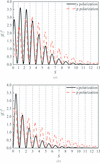Figure 7 Field intensity distribution inside optimized (a) chirped and (b) periodic Mo/Si multilayer mirrors.

Finally, we study the effect of roughness on the performances of phase retarders based on chirped multilayer structures. A commonly used roughness model (Garakhin et al., 2017) is employed, which assumes a 1.2 nm Mo-on-Si boundary roughness and 0.6 nm Si-on-Mo boundary roughness. We used a chirped multilayer structure based phase retarder (N = 13, Dc = 14.7 nm, E = 90 eV), detailed in Table 2, to investigate the effect of roughness. The curves of the phase retardation (Δφ) and reflectivity R for s- and p-polarized light (Rs and Rp) with varying incidence angle (θ) are shown in Fig. 8. When the roughness effect is considered, the curves of phase retardation and the reflectivity all drop down slightly compared with those that neglect roughness. We list the main parameter values at the incident angle of 60° for neglecting and including roughness in Table 3. It is found that the difference in phase retardation is only 0.28°, while the corresponding P (output efficiency) is reduced by 3.15%. As a result, the roughness of layers can decrease reflectivity, but has a minimal effect on the phase retardation, which indicates good stability and applicability of phase retarders based on chirp multilayer structures.

 Table 3Effect of roughness (1.2 nm Mo-on-Si and 0.6 nm Si-on-Mo boundary roughness) on the main parameters of phase retardation and output efficiency
 Δφ is the phase retardation, Rs and Rp represent the reflectivity for s- and p-polarized lights, respectively, and P is the output efficiency.
Structural defects Δφ (°) Rs Rp P
Neglecting roughness 89.95 0.4765 0.1009 0.1665
Including roughness 89.67 0.3956 0.0814 0.1350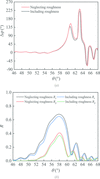Figure 8 Effect of roughness (1.2 nm Mo-on-Si and 0.6 nm Si-on-Mo boundary roughness) on (a) phase retardation and (b) reflectivity of s- and p-polarized light.

### 4. Conclusions

This paper proposes a design method of EUV phase retarders based on linearly chirped Mo/Si multilayer mirrors. The reflective phase retardation of a chirped Mo/Si multilayer with varying structural parameters such as thickness and bilayer number are investigated. The results show that, as the thickness variation of the linearly chirped multilayer increases, the reflective phase retardation between s- and p-polarized light increases at first and then reaches its maximum value. In addition, the maximum phase retardation increases with increasing bilayer number. When the bilayer number of a multilayer increases from 2 to 20, the maximum reflective phase retardation increases from 5.97° to 245.10°. The reflectivity of the linearly chirped Mo/Si multilayer is also investigated, and when the thickness variation of a linearly chirped multilayer increases, its reflectivity decreases at first. It seems that there is a trade-off between phase retardation and reflectivity for the linearly chirped multilayer. Furthermore, the maximum phase retardation of a chirped Mo/Si multilayer for different photon energies of incident light was also studied. We demonstrated that the linearly chirped mirror can achieve higher phase retardation at a smaller photon energy. We also studied the field intensity distribution inside optimized chirped Mo/Si multilayer mirrors. We observed that the light travels further inside a chirped mirror than a periodic one, and encounters more interfaces determined to be the origin of phase retardation improvement. This research opens the door for phase control of EUV sources, which is very important for the generation and application of circularly polarized EUV light sources and the complete polarization state analysis of synchrotron radiation.

### Acknowledgements

The authors declare no conflicts of interest.

### Funding information

We gratefully acknowledge scientific support by the National Natural Science Foundation of China (grant Nos. 61805007; 11547241).

### ReferencesJOURNAL OFSYNCHROTRONRADIATION